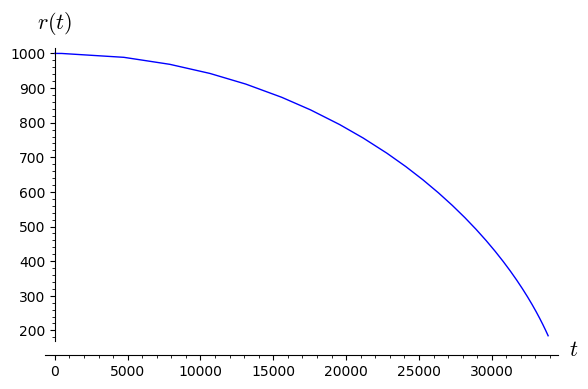# Revision history [back]

You can do it by setting the attribute terminal to True in the event function of solve_ivp:

from scipy.integrate import solve_ivp
def rhs(t, (r, v)):     # v stands for dr/dt
return (v, -1/r^2)
r_min = 200.
return 0 if r < r_min else 1
t0, r0, v0 = 0, 1000., 0  # initial conditions
t_max = 50000
sol = solve_ivp(rhs, (t0, t_max), (r0, v0), events=small_radius, rtol=1e-8)
sol.message


gives

'A termination event occurred.'


You can plot the obtained solution by

line(zip(sol.t, sol.y), axes_labels=[r'$t$', r'$r(t)$'])


and check that it indeed stops at r=r_min:You can do it by setting the attribute terminal to True in the event function of solve_ivp:

from scipy.integrate import solve_ivp
def rhs(t, (r, v)):     # v stands for dr/dt
return (v, -1/r^2)
r_min = 200.
return 0 if r < r_min else 1
t0, r0, v0 = 0, 1000., 0  # initial conditions
t_max = 50000
sol = solve_ivp(rhs, (t0, t_max), (r0, v0), events=small_radius, rtol=1e-8)
sol.message


gives

'A termination event occurred.'


You can plot the obtained solution by

line(zip(sol.t, sol.y), axes_labels=[r'$t$', r'$r(t)$'])


and check that it indeed stops at r=r_min:For more details, see the documentation of solve_ivp.

You can do it by setting the attribute terminal to True in the event function of solve_ivp:

from scipy.integrate import solve_ivp
def rhs(t, (r, v)):     # v stands for dr/dt
r'' = -1/r^2 rewritten as the system
return (v, -1/r^2)
-1/r^2)  # r' = v, v' = -1/r^2
r_min = 200.
return 0 if r < r_min else 1
t0, r0, v0 = 0, 1000., 0  # initial conditions
t_max = 50000
sol = solve_ivp(rhs, (t0, t_max), (r0, v0), events=small_radius, rtol=1e-8)
sol.message


gives

'A termination event occurred.'


You can plot the obtained solution by

line(zip(sol.t, sol.y), axes_labels=[r'$t$', r'$r(t)$'])


and check that it indeed stops at r=r_min:For more details, see the documentation of solve_ivp.